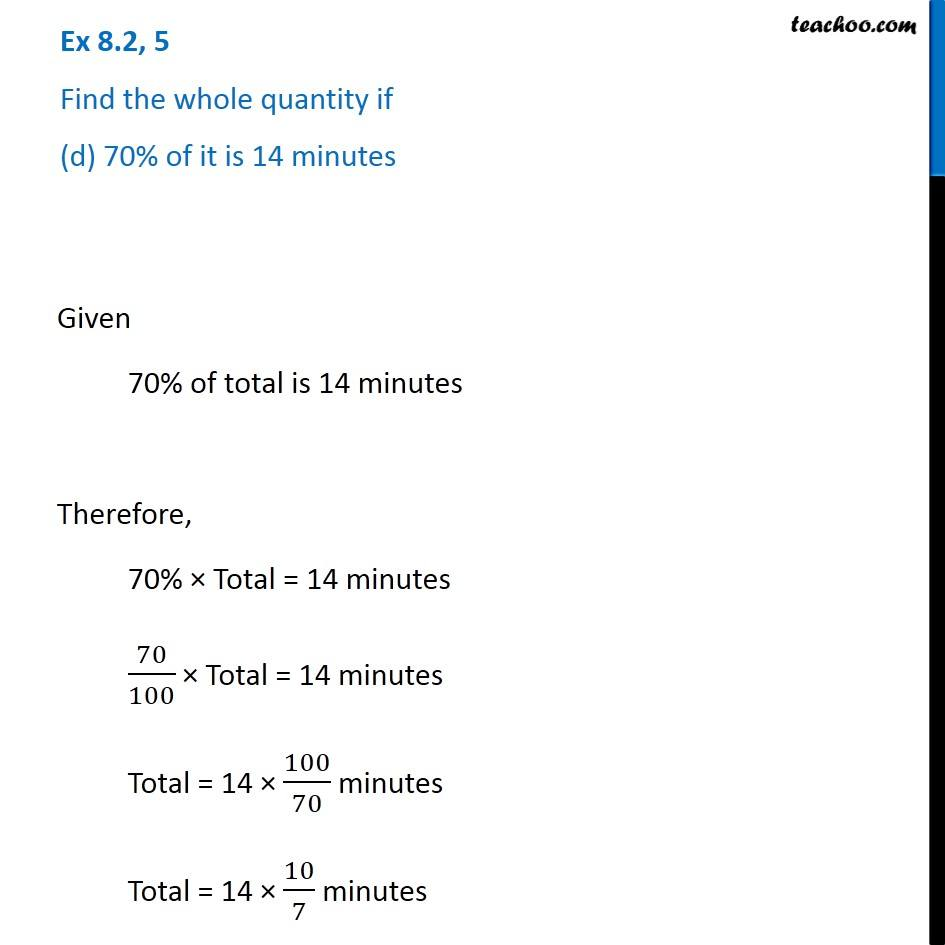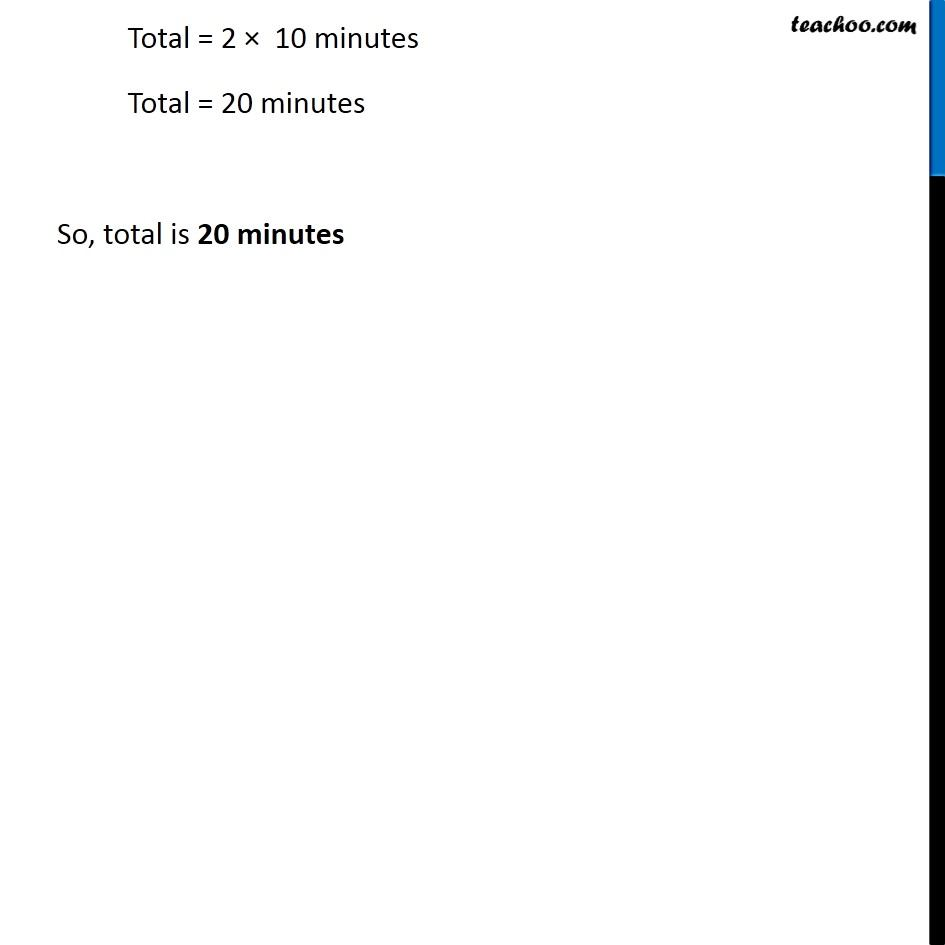1. Chapter 8 Class 7 Comparing Quantities
2. Serial order wise
3. Ex 8.2

Transcript

Ex 8.2, 5 Find the whole quantity if (d) 70% of it is 14 minutes Given 70% of total is 14 minutes Therefore, 70% × Total = 14 minutes 70/100 × Total = 14 minutes Total = 14 × 100/70 minutes Total = 14 × 10/7 minutes Total = 2 × 10 minutes Total = 20 minutes So, total is 20 minutes